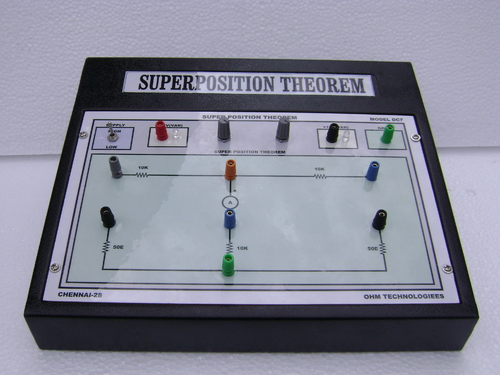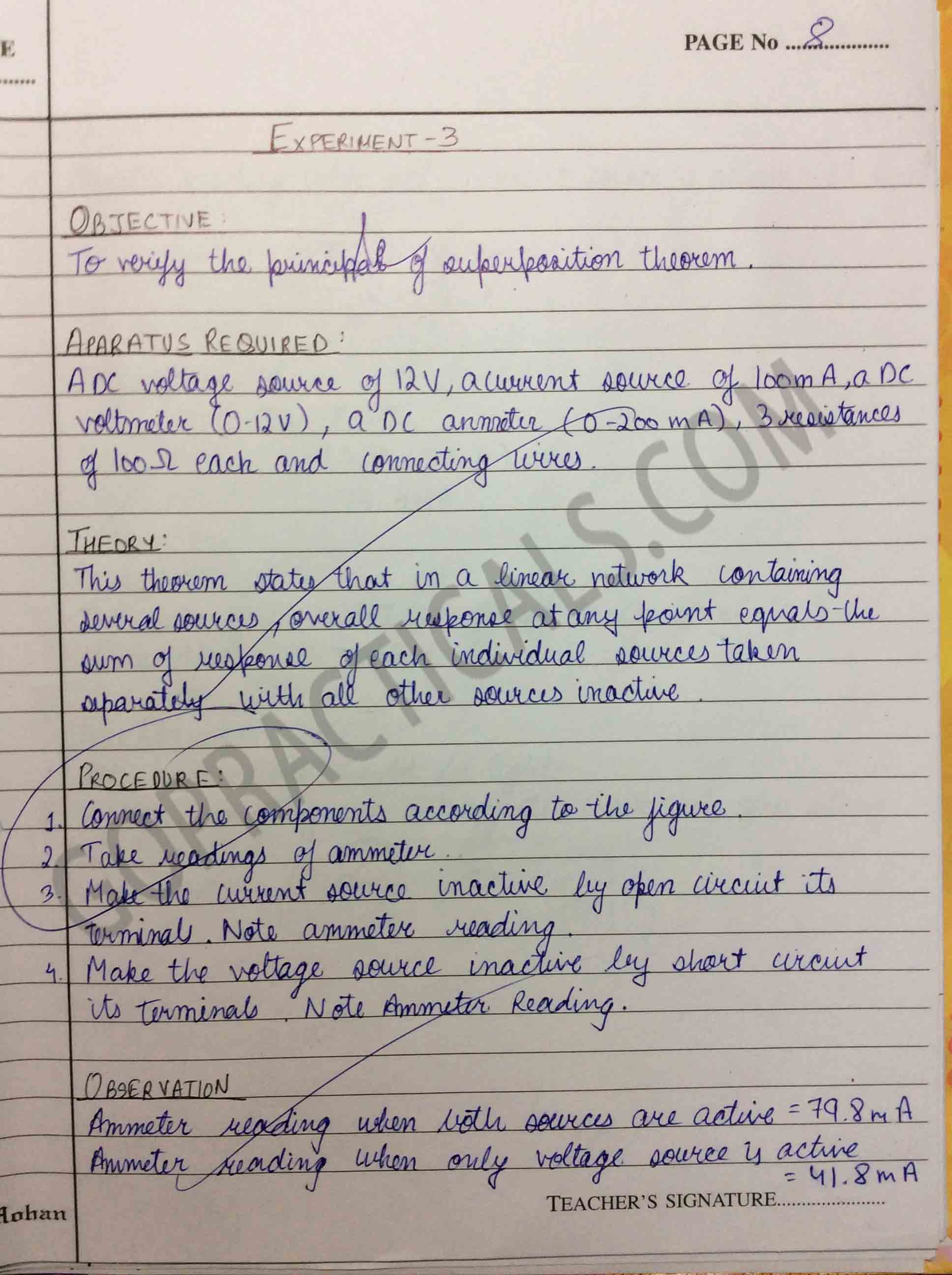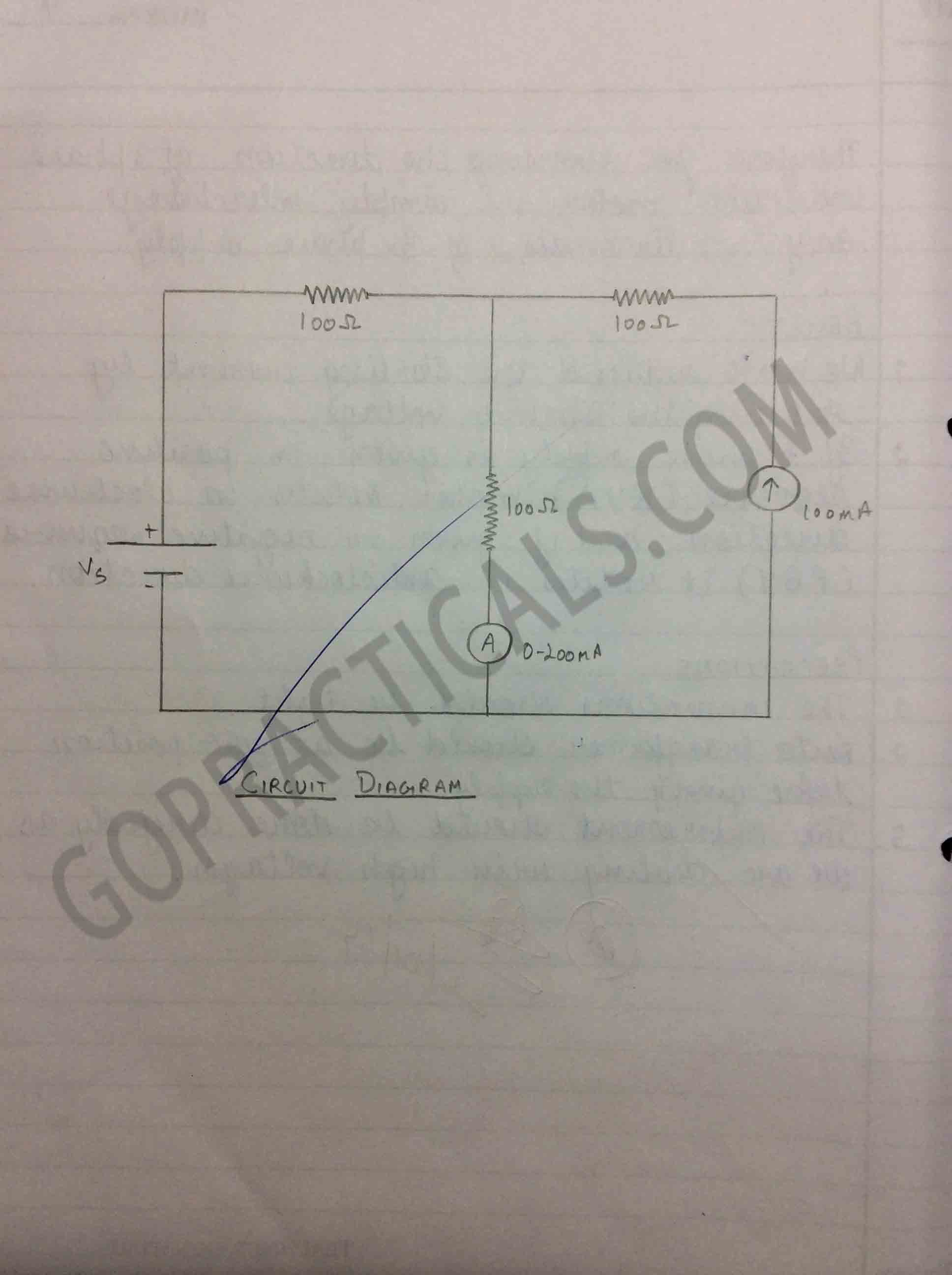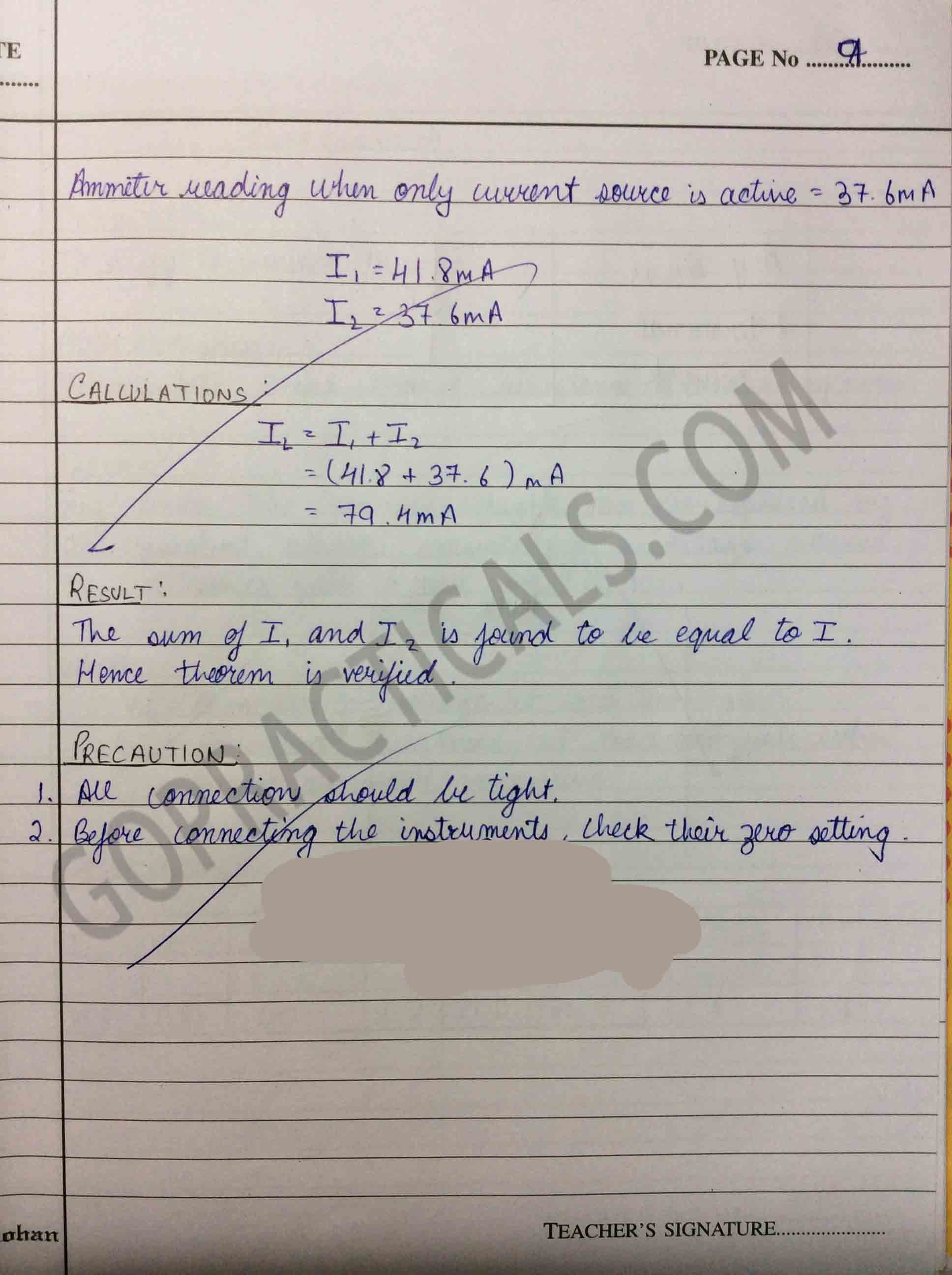Superposition Theorem states that a circuit can be analysed with only one source of power at a time, the corresponding component voltages and currents algebraically added to find out what they’ll do with all power sources in effect. To negate all but one power source for analysis, replace any source of voltage (batteries) with a wire; replace any current source with an open (break). In this practical we will learn to verify the Superposition Theorem.

Do Check Out – Thévenin’s Theorem## Practical on verification of Superposition Theorem## Watch this Video to know about Superposition Theorem

Thanks for viewing our post, hope you liked it and if you think we are helping you then do share our posts.

Verification of Principle of Superposition Theorem – Electrical Practical